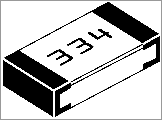Surface Mount Resistors

All SM resistors conform to a 3-digit or 4-digit code. But there are a number of codes, according to the tolerance of the resistor. It's getting very complicated.
Here is a basic 3-digit SM resistor:A 330k SM resistor

The first two digits represent the two digits in the answer. The third digit represents the number of zero's you must place after the two digits. The answer will be OHMS. For example: 334 is written 33 0 000. This is written 330,000 ohms. The comma can be replaced by the letter "k". The final answer is: 330k.
222 = 22 00 = 2,200 = 2k2
473 = 47 000  = 47,000 = 47k
105 = 10 00000 = 1,000,000 = 1M = one million ohms
There is one trick you have to remember. Resistances less than 100 ohms are written: 100, 220, 470. These are 10 and NO zero's = 10 ohms = 10R
or 22 and no zero's = 22R  or 47 and no zero's = 47R.  Sometimes the resistor is marked: 10, 22 and 47 to prevent a mistake.

Remember:
R = ohms
k = kilo ohms = 1,000 ohms
M = Meg = 1,000,000 ohms
The 3 letters (R, k and M) are put in place of the decimal point. This way you cannot make a mistake when reading a value of resistance.

THE COMPLETE RANGE OF SM RESISTOR
MARKINGS

Click to see the complete range of SM resistor markings for 3-digit code:

Click to see the complete range of SM resistor markings for 4-digit code:

0000 is a value on a surface-mount resistor. It is a zero-ohm LINK!
Resistances less than 10 ohms have  'R' to indicate the position of the decimal point.
Here are some examples:

 Three Digit Examples Four Digit Examples 330 is 33 ohms - not 330 ohms 1000 is 100 ohms - not 1000 ohms 221 is 220 ohms 4992 is 49 900 ohms, or 49k9 683 is 68 000 ohms, or 68k 1623 is 162 000 ohms, or 162k 105 is 1 000 000 ohms, or 1M 0R56 or R56 is 0.56 ohms 8R2 is 8.2 ohms

A new coding system has appeared on 1% types. This is known as the EIA-96 marking method. It consists of a three-character code. The first two digits signify the 3 significant digits of the resistor value, using the lookup table below. The third character - a letter - signifies the multiplier.

 code value code value code value code value code value code value 01 100 17 147 33 215 49 316 65 464 81 681 02 102 18 150 34 221 50 324 66 475 82 698 03 105 19 154 35 226 51 332 67 487 83 715 04 107 20 158 36 232 52 340 68 499 84 732 05 110 21 162 37 237 53 348 69 511 85 750 06 113 22 165 38 243 54 357 70 523 86 768 07 115 23 169 39 249 55 365 71 536 87 787 08 118 24 174 40 255 56 374 72 549 88 806 09 121 25 178 41 261 57 383 73 562 89 825 10 124 26 182 42 267 58 392 74 576 90 845 11 127 27 187 43 274 59 402 75 590 91 866 12 130 28 191 44 280 60 412 76 604 92 887 13 133 29 196 45 287 61 422 77 619 93 909 14 137 30 200 46 294 62 432 78 634 94 931 15 140 31 205 47 301 63 442 79 649 95 953 16 143 32 210 48 309 64 453 80 665 96 976

The multiplier letters are as follows:

 letter mult letter mult F 100000 B 10 E 10000 A 1 D 1000 X or S 0.1 C 100 Y or R 0.01

22A is a 165 ohm resistor, 68C is a 49900 ohm (49k9) and 43E a 2740000 (2M74). This marking scheme applies to 1% resistors only.

A similar arrangement can be used for 2% and 5% tolerance types. The multiplier letters are identical to 1% ones, but occur before the number code and the following code is used:

 2% 5% code value code value code value code value 01 100 13 330 25 100 37 330 02 110 14 360 26 110 38 360 03 120 15 390 27 120 39 390 04 130 16 430 28 130 40 430 05 150 17 470 29 150 41 470 06 160 18 510 30 160 42 510 07 180 19 560 31 180 43 560 08 200 20 620 32 200 44 620 09 220 21 680 33 220 45 680 10 240 22 750 34 240 46 750 11 270 23 820 35 270 47 820 12 300 24 910 36 300 48 910

With this arrangement, C31 is 5%, 18000 ohm (18k), and D18 is 510000 ohms (510k) 2% tolerance.
Always check with an ohm-meter (a multimeter) to make sure.

Chip resistors come in the following styles and ratings:
Style:
0402, 0603, 0805, 1206, 1210, 2010, 2512, 3616, 4022
Power Rating: 0402(1/16W), 0603(1/10W), 0805(1/8W), 1206(1/4W), 1210(1/3W), 2010(3/4W), 2512(1W), 3616(2W), 4022(3W)
Tolerance: 0.1%, 0.5%, 1%, 5%
Temperature Coefficient: 25ppm 50ppm 100ppm